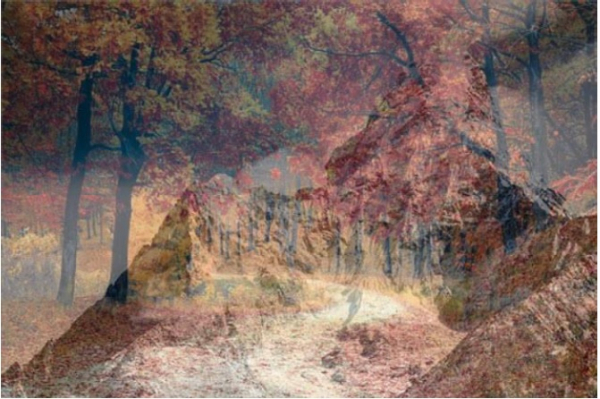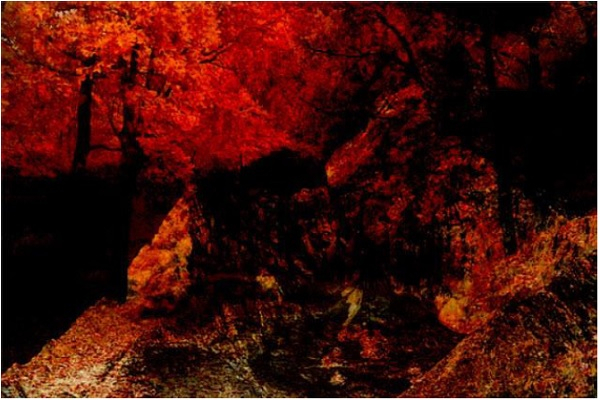# Arithmetic Operations on Images using OpenCV in Python

In this tutorial, we are going to learn about the arithmetic operations on Images using OpenCV. We can apply operations like addition, subtraction, Bitwise Operations, etc.., Let's see how we can perform operations on images.

We need the OpenCV module to perform operations on images. Install the OpenCV module using the following command in the terminal or command line.

pip install opencv-python==4.1.1.26

If you run the above command, you will get the following successful message.

Collecting opencv-python==4.1.1.26
6221dd8d05119b9782d4c2b1d1482e22b5f5e/opencv_python-4.1.1.26-cp37-cp37m-win_amd64.w
hl (39.0MB)
Requirement already satisfied: numpy>=1.14.5 in c:\users\hafeezulkareem\anaconda3\l
ib\site-packages (from opencv-python==4.1.1.26) (1.16.2)
Installing collected packages: opencv-python
Successfully installed opencv-python-4.1.1.26

We can add two images using the cv2.addWeighted(). It takes five arguments, two images, and the weight of the final image from both and the light value for the final image.

#### image_one#### image_TwoNow we are going to add those two images into one image.

### Example

# importing cv2 module
import cv2
# reading the images and storing in variables
result_image = cv2.addWeighted(image_one, 0.5, image_two, 0.5, 0)
# displaying the final image
cv2.imshow('Final Image', result_image)
# deallocating the memory
if cv2.waitKey(0) & 0xff == 27:
cv2.destroyAllWindows()

### Output

Final Image## Subtraction

We have a method called cv2.substract(image_one, image_two) to perform subtraction on two images. We are going to use the same images as an addition. Let's see the code.

### Example

# importing cv2 module
import cv2
# reading the images and storing in variables
# substracting two images
result_image = cv2.subtract(image_one, image_two)
# displaying the final image
cv2.imshow('Final Image', result_image)
# deallocating the memory
if cv2.waitKey(0) & 0xff == 27:
cv2.destroyAllWindows()

### Output

Final Image## Conclusion

If you have any doubts regarding the tutorial, mention them in the comment section.